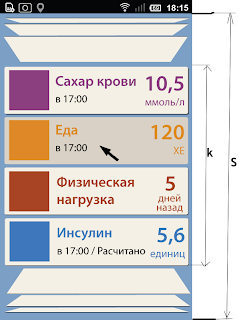## November 16, 2011

### Coverflow

Here is theory how to implement coverflow scrollingGiven:

1. S - height of container
2. k - height of zone where badges are shown without folding (k < S)
3. h - height of a badge (h < k)
4. n - amount of badges
Problem:
Find top (y?) position of each t badge (t in [0..(n-1)]) if the list scrolled by q factor (q in [0;n*h-k] where 0 - means showing top badges without folding, n*h-k - means showing bottom badges without folding)

Solution:
We need an interpolator function f(x) which is
1. Is defined in [0;1] and rising
2. f(0) = 0
3. f(1) = 1
This function defines how badges are folded
Good example of such function is f(x) = x*x

Calculation
v = n*h-k
z = t*h
p = q/v
alpha = (S-k)/(f(p)+f(1-p))

Then
1. y = alpha * f(z/v) if z < q
2.  y = z + alpha * f(p) - vp if z >= q and z <= q+k
3. y = S - alpha * f((n*h-z)/v) if z > q + k

## November 10, 2011

### Printing from an Android application

Printing Directly to a Network Printer
Send 2 Printer - Payable Android app which provides api for 3rd party apps for printing